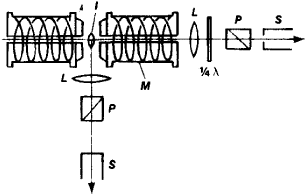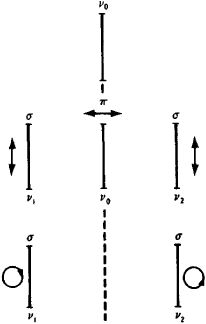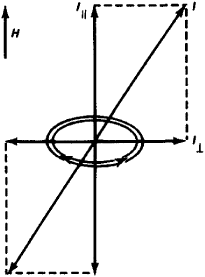# Zeeman effect

Also found in: Dictionary, Thesaurus, Medical, Wikipedia.
Related to Zeeman effect: Stark effect, Anomalous zeeman effect

## Zeeman effect,

splitting of a single spectral line (see spectrumspectrum,
arrangement or display of light or other form of radiation separated according to wavelength, frequency, energy, or some other property. Beams of charged particles can be separated into a spectrum according to mass in a mass spectrometer (see mass spectrograph).
) into a group of closely spaced lines when the substance producing the single line is subjected to a uniform magnetic field. The effect was discovered in 1896 by the Dutch physicist Pieter Zeeman. In the so-called normal Zeeman effect, the spectral line corresponding to the original frequency of the light (in the absence of the magnetic field) appears with two other lines arranged symmetrically on either side of the original line. In the anomalous Zeeman effect (which is actually more common than the normal effect), several lines appear, forming a complex pattern. The normal Zeeman effect was successfully explained by H. A. Lorentz using the laws of classical physics (Zeeman and Lorentz shared the 1902 Nobel Prize in Physics). The anomalous Zeeman effect could not be explained using classical physics; the development of the quantum theoryquantum theory,
modern physical theory concerned with the emission and absorption of energy by matter and with the motion of material particles; the quantum theory and the theory of relativity together form the theoretical basis of modern physics.
and the discovery of the electron's intrinsic spin led to a satisfactory explanation. According to the quantum theory all spectral lines arise from transitions of electrons between different allowed energy levels within the atom, the frequency of the spectral line being proportional to the energy difference between the initial and final levels. Because of its intrinsic spin, the electron has a magnetic field associated with it. When an external magnetic field is applied, the electron's magnetic field may assume only certain alignments. Slight differences in energy are associated with these different orientations, so that what was once a single energy level becomes three or more. Practical applications based on the Zeeman effect include spectral analysis and measurement of magnetic field strength. Since the separation of the components of the spectral line is proportional to the field strength, the Zeeman effect is particularly useful where the magnetic field cannot be measured by more direct methods.

## Zeeman effect

A splitting of spectral lines when the light source being studied is placed in a magnetic field. Discovered by P. Zeeman in 1896, the effect furnishes information of prime importance in the analysis of spectra. Each kind of spectral term has its characteristic mode of splitting, and the types of terms are most definitely identified by this property, Furthermore, the effect allows an evaluation of the ratio of charge to mass of the electron and an evaluation of its precise magnetic moment.

The normal Zeeman effect is a splitting into two or three lines, depending on the direction of observation, as shown in the illustration. The light of these components is polarized in ways indicated in the illustration. The normal effect is observed for all lines belonging to singlet systems, those for which the spin quantum number S = 0. The change of frequency of the shifted components can be evaluated on classical electromagnetic principles.

The anomalous Zeeman effect is a more complicated type of line splitting, so named because it did not agree with the predictions of classical theory. It occurs for any spectral line arising from a combination of terms of multiplicity greater than one. Since multiplicity in spectral lines is caused by the presence of a resultant spin vector S of the electrons, the anomalous effect must be attributed to a nonclassical magnetic behavior of the electron spin.

The quadratic Zeeman effect, which depends on the square of the field strength, is of two kinds. The first results from second-order terms, and the second from the diamagnetic reaction of the electron when revolving in large orbits.

The inverse Zeeman effect is the Zeeman effect of absorption lines. It is closely related to the Faraday effect, the rotation of plane-polarized light by matter situated in a magnetic field. See Faraday effect

The Zeeman effect in molecules is, in general, so small as to be unobservable, even for molecules which have a permanent magnetic moment. An exception occurs for some light molecules where the magnetic moment is coupled so lightly to the frame of the molecule that it can orient itself freely in the magnetic field just as for atoms.

A clear Zeeman effect also can be observed in many crystals with sharp spectrum lines in absorption or fluorescence. Such crystals are found particularly among rare-earth salts.

The magnetic moment of the nucleus causes a Zeeman splitting in atomic spectra which is of an order of magnitude a thousand times smaller than the ordinary Zeeman effect. This Zeeman effect of the hyperfine structure usually is modified by a nuclear Paschen-Back effect. See Paschen-Back effect

## Zeeman Effect

the splitting of spectral lines under the influence of a magnetic field. It was discovered in 1896 by P. Zeeman while studying the luminosity of sodium vapor in a magnetic field. To observe the Zeeman effect, a light source emitting a line spectrum is placed between the poles of a strong electromagnet (Figure 1), whereupon each spectralFigure 1. Diagram of observation of the Zeeman effect. The source of the line spectrum / lies between the poles of an electromagnet M, whose core is drilled out to allow observation along the field. The lenses L, the polaroids P, and a plate 1/4 of a wavelength thick are used to determine the type of polarization; S is a spectroscope.

line splits into several components. The splitting is very small (several tenths of an angstrom for magnetic fields of —20 kilo-oersteds); therefore, high-resolution spectral devices are used to observe the Zeeman effect.

All components of Zeeman splitting are polarized. The splitting pattern and the polarization of the components depend on the direction of observation. In the simplest case (Figure 2), three lines are observed in a direction perpendicular to that of the magnetic field (the transverse Zeeman effect): an undisplaced π-component, which is polarized in the direction of the field, and two σ-components, which is polarized in the direction of the field, and two π-components, which are located symmetrically with respect to the Trcomponent and polarized perpendicular to the field. Only the σ-components, which in this case are polarized about a circle, remain upon observation in the direction of the field (the longitudinal Zeeman effect).

In 1897, H. Lorentz gave the first explanation of the Zeeman effect. He considered an electron in an atomFigure 2. Normal Zeeman effect: (top) without a field, the line v0 is not polarized; (center) with transverse observation in a magnetic field, a triplet having the frequencies v1, v0, and v2 the lines are linearly polarized (the direction of polarization is indicated by the arrows); (bottom) with longitudinal observation, a doublet having the frequencies v1 and v2, and the lines are polarized about a circle in a plane perpendicular to the magnetic field; v1 = v0 + >v1v2 = v0 — Δv.

as a harmonic oscillator of frequency v0, radiating a spectral line of this frequency in the absence of an external field. In a homogeneous external magnetic field H, the motion of a linearly oscillating electron may be divided into a linear oscillation along the direction of the field and two rotary oscillations (with opposite directions of rotation) in a plane perpendicular to H (Figure 3). The field HFigure 3. Resolution of the harmonic oscillator / into the linear oscillators / along the direction of the field and / perpendicular to the field. The oscillator / breaks down into two circular oscillators rotating in opposite directions.

does not act on the linear oscillation, and its frequency remains equal to v0; the frequencies of the rotary components change, since an electron in a magnetic field gains additional rotation around the direction of the magnetic field with a frequency Δv = ¼π(e/me)H, where e/me, is the ratio of the charge of the electron to its mass. The frequencies of the oscillations become equal to v1 = v0 + Δv and v2 = v0 - Δv. Thus, the atom in the magnetic field emits three lines with frequencies v0, v1, and v2 (a Zeeman triplet). This type of splitting—the simple (or normal) Zeeman effect—occurs only for single spectral lines and in the extreme case of very strong magnetic fields (the Paschen-Back effect). A more complicated picture is generally observed: the spectral line splits into a large number of components with various values of Δv—the complex (or anomalous) Zeeman effect; the result is a spectral group of equidistant π-components and two groups of equidistant o-components that are symmetrical with respect to it.

Quantum theory gives a full explanation ot the Zeeman effect. A quantum system such as ah atom has a magnetic moment μ, which is linked to the mechanical movement of momentum M and may be oriented in the magnetic field only in a specific way. The number of possible orientations of μ is equal to the degree of degeneracy of the energy level, that is, to the number of possible states of an atom with a given energy E. In a magnetic field an additional energy ΔE corresponds to each orientation ofμ. This leads to the relaxation of degeneracy, and the level splits.

The additional energy ΔE is proportional to the magnitude of the field intensity H:

ΔE = μHH

where μH is the projection of μ in the direction of the field H.

In a magnetic field, μH assumes discrete values equal to gμBm, where g is the Landé factor, μB is a Bohr magneton, and m is a magnetic quantum number (m =J; J - 1 , . . . - J, where J is a quantum number that determines the possible values of M).

As a result, the additional energy

ΔEm = –μHH = gμBH .m

is different for different magnetic quantum numbers, and the energy level E splits into 2J + 1 equally spaced Zeeman sublevels. The distance between neighboring sublevels E+1 is equal to

δ = ΔEm+1 — ΔEm = gμBH = g ΔE0

where ΔE0 = μBH is the extent of so-called normal splitting.

If gi = g2 for levels E1 and E2, between which a quantum transition takes place, then the splitting of the spectral line in the magnetic field is a Zeeman triplet. If g1g2, then an anomalous Zeeman effect results.

The study of the pattern of the Zeeman effect makes it possible to determine the characteristics of the energy levels of various atoms. In addition to quantum transitions between Zeeman sublevels of various energy levels (the Zeeman effect in spectral lines), magnetic quantum transitions can be observed between the Zeeman sublevels of a single level. Such transitions take place upon exposure to radiation of frequency v =δ/h (where h is Planck’s constant). In ordinary magnetic fields the frequencies of such transitions correspond to the superhigh-frequency band. This leads to the selective absorption of radio waves, which can be observed in paramagnetic substances placed in a permanent magnetic field.

The Zeeman effect is also observed in molecular spectra, but they are much more difficult to decode than atomic spectra. In addition, observation of the Zeeman effect in molecular spectra presents great experimental difficulties because of the complexity of the splitting and of the overlap of molecular spectral bands. The Zeeman effect can also be observed in the spectra of crystals (especially in absorption spectra).

The Zeeman effect is used not only in spectroscopy to investigate the fine structure of matter but also in quantum electronics devices and to measure magnetic fields under laboratory conditions and the magnetic fields of objects in space.

### REFERENCES

Landsberg, G. S. Optika, 4th ed. Moscow, 1957. (Obshchii kurs fiziki, vol. 3.)
El’iashevich, M. A.Atomnaia i molekuliarnaia spektroskopiia. Moscow, 1962.
Herzberg, G. Spektry i stroenie dvukhatomnykh molekul. Moscow, 1949. (Translated from English.)

M. A. EL’IASHEVICH

## Zeeman effect

[′zā·mən i‚fekt]
(spectroscopy)
A splitting of spectral lines in the radiation emitted by atoms or molecules in a static magnetic field.
References in periodicals archive ?
Due to the linearity of the Zeeman effect as previously discussed, the line-of-sight integration can be modeled as
The use of Zeeman effect background correction with 3-field modulation for extending the dynamic measuring range in graphite-furnace AAS has proved successful.
However, as a test of a specific causal type of mechanism it is not always reliable since the effect can be smeared out or eliminated by the presence of multiple gas isotopes, the Zeeman effect, as well as others.
1:30 QUALITATIVE ANALYSIS OF THE PACIFIC SCIENCE PHYSICS EQUIPMENT ZEEMAN EFFECT APPARATUS, David Bolding*, David Mertins*, Robert Moore, Jr.
At the NFL, the sample aliquots were acid digested and analyzed by GFAAS, with Zeeman effect background correction and using the standard additions method with a matrix modifier [Pd + Mg[([NO.sub.3]).sub.2]].
This Zeeman effect could be used to study the fine details of atomic structure and to yield information on the structure of stars as well.
the Compton effect, the Zeeman effect, the Josephson effect, etc.
Tenders are invited for E-procurement scheme for zeeman effect experimental set-up
Tenders are invited for Supply And Installationof Zeeman Effect Setup
The Measuring Principle Of The Apparatus Resides In An Optical Analysis Of The Mercury By Zeeman Effect.

Site: Follow: Share:
Open / Close